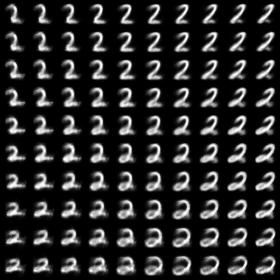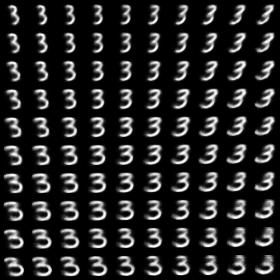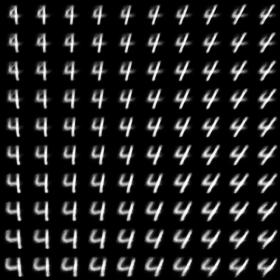# Conditional Variational Auto-Encoder for MNIST

An implementation of conditional variational auto-encoder (CVAE) for MNIST descripbed in the paper:

## Implemented Models

The paper suggests three models.

• Latent Discriminative Model (M1) : M1 is the same as in Auto-Encoding Variational Bayes, and my implementation is given at here.
• Generative Semi-Supervised model (M2) : M2 is implemented in here but with full labeled data. My concern is not classification performance of semi-supervised model, but effect of condition vector.
• Stacked model (M1+M2) : This is not implemented.

If you are interested in missing parts, please see original implementation or replications (Tensorflow) (Chainer) of it.

## Results

### Reproduce

Well trained auto-encoder must be able to reproduce input image.
Since CVAE uses additional information (labels) which is not used in VAE, CVAE shows better performance than CVAE.
The following results can be reproduced with command:

``````python run_main.py --dim_z 2
``````

### Denoising

When training, salt & pepper noise is added to input image, so that CVAE can reduce noise and restore original input image.
The following results can be reproduced with command:

``````python run_main.py --dim_z 2 --add_noise True
``````

### Conditional Generation : Learned MNIST manifold

Visualisation of handwriting styles learned by the model with 2D z-space in Figure. 1(a) in the paper.
The following results can be reproduced with command:

``````python run_main.py --dim_z 2 --PMLR True
``````
 Learned MNIST manifold with a condition of label 2 Learned MNIST manifold with a condition of label 3 Learned MNIST manifold with a condition of label 4### Conditional Generation : Analogical reasoning with random z-vectors

Analogical reasoning with generative semi-supervised models using a 2D z-space like in Figure. 1(b) in the paper.
The following results can be reproduced with command:

``````python run_main.py --dim_z 2 --PARR True
``````

The leftmost columns show images generated from 4 z-vectors. The other columns show analogical fantasies of x by the generative model, where the latent variable z of each row is set to the value inferred from the test-set image on the left by the inference network.

### Conditional Generation : Analogical reasoning with a given image

CVAE encodes a given handwritten digit image to a z-vector.
With an obtained z-vector, various images with similar style to the given image can be generated by changing label-condition.

## Usage

### Prerequisites

1. Tensorflow
2. Python packages : numpy, scipy, PIL(or Pillow), matplotlib

### Command

``````python run_main.py --dim_z <latent vector dimension>
``````

Example: `python run_main.py --dim_z 20`

### Arguments

Required :

• `--dim_z`: Dimension of latent vector. Default: `20`

Optional :

• `--results_path`: File path of output images. Default: `results`
• `--add_noise`: Boolean for adding salt & pepper noise to input image. Default: `False`
• `--n_hidden`: Number of hidden units in MLP. Default: `500`
• `--learn_rate`: Learning rate for Adam optimizer. Default: `1e-3`
• `--num_epochs`: The number of epochs to run. Default: `20`
• `--batch_size`: Batch size. Default: `128`
• `--PRR`: Boolean for plot-reproduce-result. Default: `True`
• `--PRR_n_img_x`: Number of images along x-axis. Default: `10`
• `--PRR_n_img_y`: Number of images along y-axis. Default: `10`
• `--PRR_resize_factor`: Resize factor for each displayed image. Default: `1.0`
• `--PMLR`: Boolean for plot-manifold-learning-result. Default: `False`
• `--PMLR_n_img_x`: Number of images along x-axis. Default: `10`
• `--PMLR_n_img_y`: Number of images along y-axis. Default: `10`
• `--PMLR_resize_factor`: Resize factor for each displayed image. Default: `1.0`
• `--PMLR_z_range`: Range for unifomly distributed latent vector. Default: `2.0`
• `--PMLR_n_samples`: Number of samples in order to get distribution of labeled data. Default: `5000`
• `--PARR`: Boolean for plot-analogical-reasoning-result. Default: `False`
• `--PARR_resize_factor`: Resize factor for each displayed image. Default: `1.0`
• `--PARR_z_range`: Range for unifomly distributed latent vector. Default: `2.0`

## References

Get A Weekly Email With Trending Projects For These Topics
No Spam. Unsubscribe easily at any time.
python (53,198
tensorflow (2,138
autoencoder (79
mnist (70
vae (69
variational-autoencoder (50
variational-inference (34
denoising (22